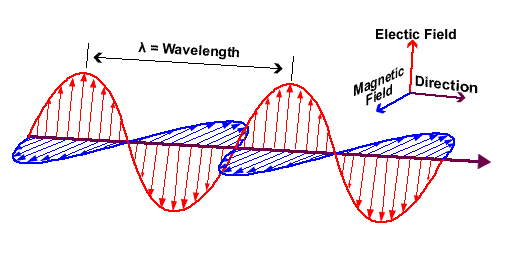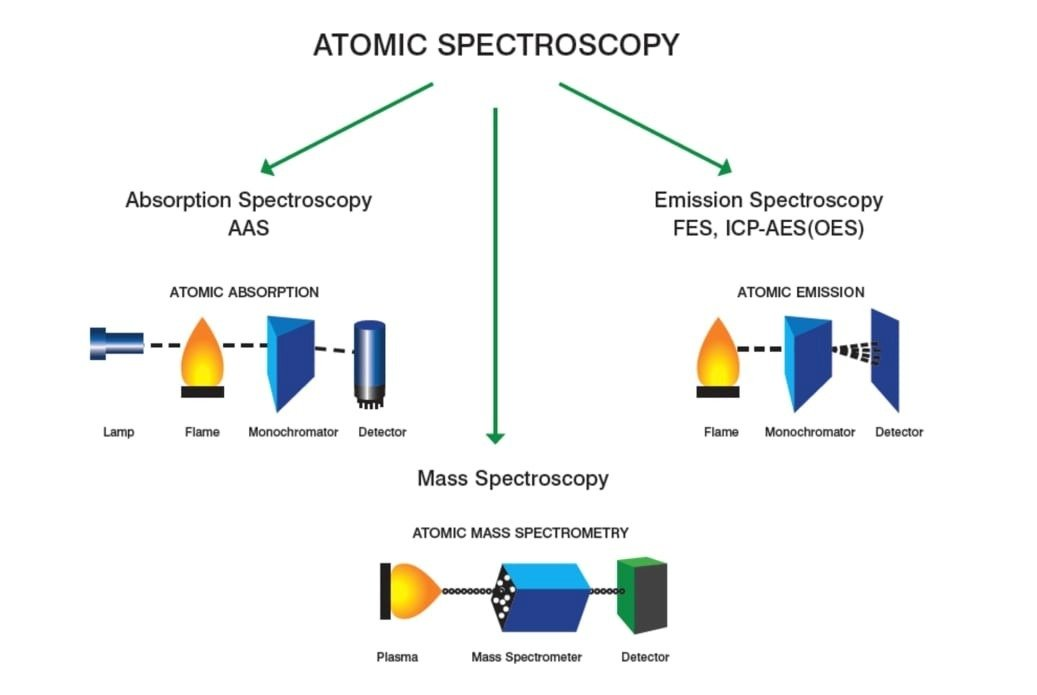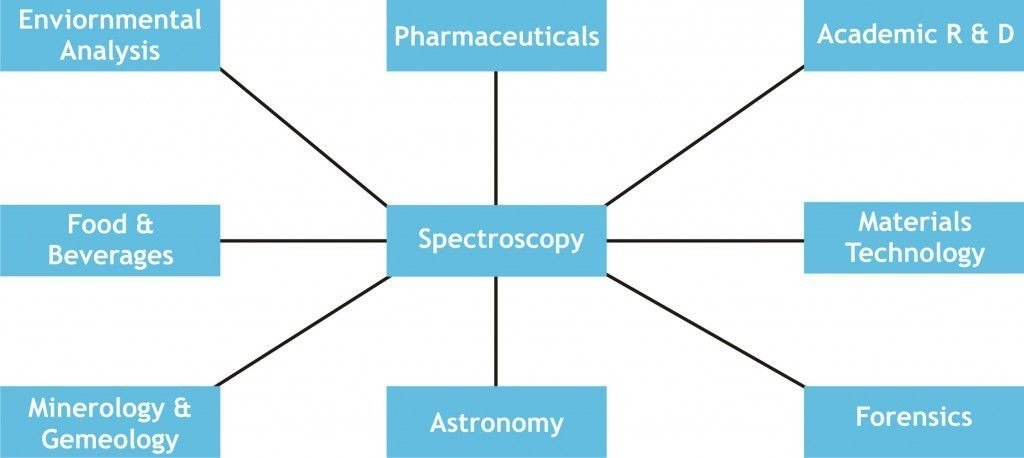# Spectroscopy: Introduction, Principles, Types and Applications

## Introduction

• Spectroscopy is a technique where we studied the interaction between matter and electromagnetic radiation.
• Matter may be ions, molecules or atoms.
• This technique employ light tends to interact with matter and exhibit some features of a sample to know much about its consistency or structure.
• The nature of the interactions in spectroscopy techniques between radiation and matter may includes- absorption, emission or scattering.

Spectroscopy techniques are two types:

1. Qualitative spectroscopy: This technique studied the radiation absorbed or emitted by an atom or molecule which will give information about its identity.
2. Quantitative spectroscopy: This technique will give information about the number of absorbing or emitting atoms or molecules by measuring the total amount of radiation.• It is a form of energy and it consists of both magnetic and electric field.
• It is composed of an electric and a perpendicular magnetic vector, each one oscillating in plane at right angles to the direction of propagation.
• Electromagnetic spectrum is mainly range from shorter wavelengths to longer wavelengths such as gamma rays.
• Wavelengths of the visible region of the spectrum is ranges between 400-700 nm.
• Blue end of the spectrum has shorter wavelengths, while red end has longer wavelengths.
• In spectrum, energy associated with the given segment is much related to the frequency and wavelength.
• Frequency (v) of the electromagnetic radiation is the number of oscillations made by wave in 1 second.
• Frequency is measured in hertz (Hz), Where, 1Hz = 1 cycle per sec.
• Wavelength (λ): It is the length of on complete wave cycle.
• Wavelength is generally measured in centimeters (cm).
• Wavelength is inversely proportional to the frequency.

The relation between wavelength and frequency is defined by:

V= c/ λ, where, c= speed of light.

E= hv = hc/ λ    where, Planck’s constant (h) = 6.6 X 10-34 joules per second.

• Wavenumber: It defines the number of completed wave cycles per distance. It is m3easuered in 1 cm-1 wavenumber is reciprocal to the wavelength.

## Types of spectroscopy

• Spectroscopy is the collective terms for diverse group of techniques which involves the interactions of electromagnetic radiation with a matter of interest are studied.
• Radiation is either get scattered, emitted or absorbed, when the radiations meets with the matter.Based on this there is three principles branches of spectroscopy:

1. Absorption spectroscopy
2. Scattering spectroscopy
3. Emission spectroscopy

Absorption spectroscopy uses the range in which a material absorbs the electromagnetic spectrum. It involves atomic absorption spectroscopy and different molecular techniques that area, such as infrared spectroscopy and radio region nuclear magnetic resonance (NMR) spectroscopy.

Emission spectroscopy uses the electromagnetic spectrum range in which a material radiates (emits). The material must consume energy first. This energy may come from several sources, such as luminescence, which defines the subsequent emission. Spectro fluorimetry includes molecular luminescence techniques.

Scattering spectroscopy tests the amount of light at specific wavelengths, incident angles, and polarization angles that a material scatter. The method of scattering is much quicker than the process of absorption/emission. Raman spectroscopy is one of the most beneficial applications of light scattering spectroscopy.

## Major types of absorption spectroscopy

 Electromagnetic radiations Spectroscopic types X- ray X-ray absorption spectroscopy UV-Visible UV/Visible absorption spectroscopy IR Infrared absorption spectroscopy Microwave Microwave absorption spectroscopy Radiowave Nuclear magnetic resonance spectroscopy

## Principles of absorption spectroscopy

• Absorption spectroscopy is based on law known as Beer-Lambert law.
• When electromagnetic radiations pass or fall onto homogeneous medium, some amount of light is reflected, some amount is absorbed and remained is transmitted.
• Absorption spectroscopy is governed by two laws i.e., Lambert’s law and Beer’s law, in the combined form it is known as Beer-Lambert law.
• Lambert’s law: It states that when monochromatic light passes through a transparent medium, the intensity of transmitted light decreases exponentially as the thickness of absorbing material increases.
• Beer’s law: It states that the intensity of transmitted monochromatic light decreases exponentially as the concentration of the absorbing substances increases.

A= log Io/ I e.c.l

A = absorbance

Io = intensity of incident light

I = intensity of light through the sample.

e = extinction coefficient or absorption coefficient.

c = concentration

l = path length (cm)

## Applications of spectroscopy• Spectroscopy used for cure monitoring of composites using optical fibers.
• It is most commonly used for estimating weathered wood exposure times using near infrared spectroscopy.
• Absorption spectroscopy is usually used both visible and infrared spectrum for Measurement of different compounds in food samples.
• For the measurement of toxic compounds in blood samples
• Non-destructive elemental analysis by X-ray fluorescence. It also used in the electronic structure research by using various types of spectroscopy.

## Reference and Sources

• https://www.researchgate.net/publication/310994699_Fundamentals_and_Techniques_of_Biophysics_and_Molecular_Biology
• https://en.wikipedia.org/wiki/Spectroscopy
• https://www.slideshare.net/LOKESHPANIGRAHI/spectroscopy-134933430